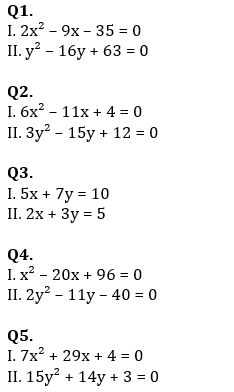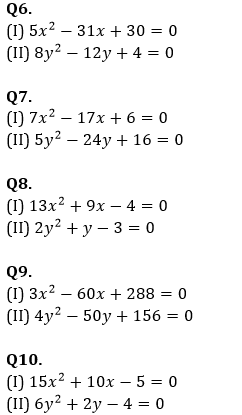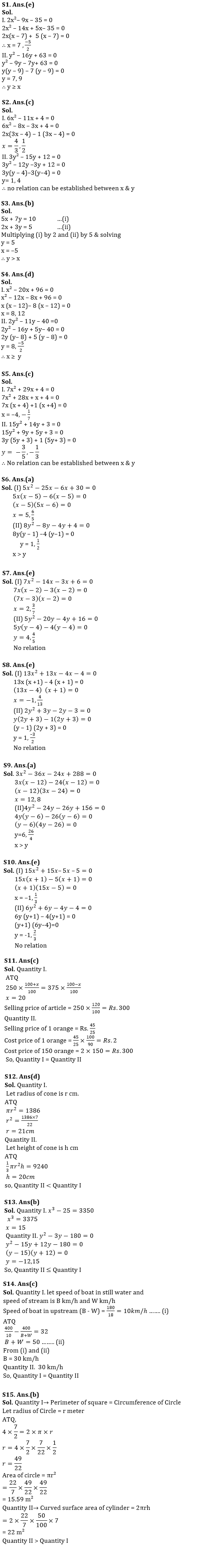Latest Banking jobs   »   Quantitative Aptitude Quiz For SBI, IBPS...

# Quantitative Aptitude Quiz For SBI, IBPS Prelims 2021- 29th March

Directions (1-5): Given below are two equations which you have to solve for the value of x and y and give answer
(a) if x >y
(b) if x < y
(c) if x = y or no relation between x and y exists
(d) if x ≥ y
(e) if x ≤ yDirections (6-10): In each of these questions, two equations (I) and (II) are given. You have to solve both the equations and give answer
(a)     if x > y
(b)     if x ≥ y
(c)     if x < y
(d)     if x ≤ y
(e)    if x = y or no relation can be established between x and y.Directions (11-15): In the following questions two quantities are given for each question. Compare the numeric value of both the quantities and answers accordingly.

Q11. Quantity I: Cost price and marked price of an article is Rs. 250 and Rs 375 respectively. Profit of x% is earned when discount of x% is given on it. Find selling price of article.
Quantity II:  A shopkeeper sold 25 orange in Rs.45 and got 10% loss. Cost price of 150 such orange.
(a) Quantity I > Quantity II
(b) Quantity II > Quantity I
(c) Quantity I = Quantity II
(d) Quantity I ≤ Quantity II
(e) Quantity II ≤ Quantity I

Q12. Volume of a cone is 9240 cm3 and area of circular base of that cone is 1386 cm2.
Quantity II: Height of cone.
(a) Quantity I ≤ Quantity II
(b) Quantity I ≥ Quantity II
(c) Quantity I = Quantity II
(d) Quantity II < Quantity I
(e) Quantity II > Quantity I

Q13. Quantity I: x³-25=3350, value of x
Quantity II: y²-3y=180, value of y
(a) Quantity I ≤ Quantity II
(b) Quantity II ≤ Quantity I
(c) Quantity I = Quantity II
(d) Quantity I > Quantity II
(e) Quantity II > Quantity I

Q14. Time taken by boat to cover 400 km in upstream is 32 hours more than the time taken by boat to cover 400 km in downstream and the total time taken by boat to cover 180 km in upstream is 18 hours.

Quantity I: Speed of boat in still water.
Quantity II:  30 km/hr.
(a) Quantity I > Quantity II
(b) Quantity II > Quantity I
(c) Quantity I = Quantity II
(d) Quantity I ≤ Quantity II
(e) Quantity II ≤ Quantity I

Q15. Quantity I:– A square of side 7/2 meter is bent into form of a circle. Area of circle.
Quantity II:– A cylinder of height 7 meter and radius of base is 50 centimeter curved surface area of cylinder is.
(a) Quantity I > Quantity II
(b) Quantity II > Quantity I
(c) Quantity I = Quantity II
(d) Quantity I ≤ Quantity II
(e) Quantity II ≤ Quantity I

Practice More Questions of Quantitative Aptitude for Competitive Exams:

###### Study Plan for IBPS and SBI Exams 2021

SolutionsPractice with Online Test Series for SBI and IBPS Prelims 2021:×

Thank You, Your details have been submitted we will get back to you.Join India's largest learning destination

What You Will get ?

•Job Alerts
•Daily Quizzes
•Subject-Wise Quizzes
•Current Affairs
•Previous year question papers
•Doubt Solving session

ORJoin India's largest learning destination

What You Will get ?

•Job Alerts
•Daily Quizzes
•Subject-Wise Quizzes
•Current Affairs
•Previous year question papers
•Doubt Solving session

ORJoin India's largest learning destination

What You Will get ?

•Job Alerts
•Daily Quizzes
•Subject-Wise Quizzes
•Current Affairs
•Previous year question papers
•Doubt Solving session

Enter the email address associated with your account, and we'll email you an OTP to verify it's you.Join India's largest learning destination

What You Will get ?

•Job Alerts
•Daily Quizzes
•Subject-Wise Quizzes
•Current Affairs
•Previous year question papers
•Doubt Solving session

Enter OTP

Please enter the OTP sent to
/6

Did not recive OTP?

Resend in 60sJoin India's largest learning destination

What You Will get ?

•Job Alerts
•Daily Quizzes
•Subject-Wise Quizzes
•Current Affairs
•Previous year question papers
•Doubt Solving sessionJoin India's largest learning destination

What You Will get ?

•Job Alerts
•Daily Quizzes
•Subject-Wise Quizzes
•Current Affairs
•Previous year question papers
•Doubt Solving session

Almost there

+91Join India's largest learning destination

What You Will get ?

•Job Alerts
•Daily Quizzes
•Subject-Wise Quizzes
•Current Affairs
•Previous year question papers
•Doubt Solving session

Enter OTP

Please enter the OTP sent to Edit Number

Did not recive OTP?

Resend 60

By skipping this step you will not recieve any free content avalaible on adda247, also you will miss onto notification and job alerts

Are you sure you want to skip this step?

By skipping this step you will not recieve any free content avalaible on adda247, also you will miss onto notification and job alerts

Are you sure you want to skip this step?